﻿ 基于双目视觉的人脸三维重建
«上一篇文章快速检索 高级检索

 智能系统学报2018, Vol. 13Issue (4): 534-542  DOI: 10.11992/tis.2017010200

### 引用本文LIN Qin, LI Weijun, DONG Xiaoli, et al. Face reconstruction based on binocular stereo vision[J]. CAAI Transactions on Intelligent Systems, 2018, 13(4): 534-542. DOI: 10.11992/tis.201701020.### 文章历史

1. 中国科学院半导体研究所 高速电路与神经网络实验室，北京 100083;
2. 中国科学院大学 电子学院，北京 100029;
3. 认知计算技术威富联合实验室，北京 100083

Face reconstruction based on binocular stereo vision
LIN Qin1,2,3, LI Weijun1,2,3, DONG Xiaoli1,2,3, NING Xin1,2,3, CHEN Peng1,2,3
1. Laboratory of Artificial Neural Networks and High-peed Circuits, Institute of Semiconductors, Chinese Academy of Sciences, Beijing 100083, China;
2. School of Microelectronics, University of Chinese Academy of Sciences, Beijing 100029, China;
3. Cognitive Computing Technology Wei Fu Joint Lab, Beijing 100083, China
Abstract: In this paper, we propose a binocular stereo algorithm called PatchMatch for generating a 3D dense point cloud of the human face. The proposed algorithm optimizes a local stereo matching method, also known as PatchMatch, which combines topological information of the human face with a local optimization algorithm for stereo vision and requires neither expensive equipment nor generic face models. With this method, by applying a non-contact binocular vision selection technology, face images at both left and right visual angles are obtained. We use an ensemble of regression trees (ERT) algorithm to position key points of a face image and estimate the sparse disparity of facial landmarks. Then, we use a linear interpolation method to make a preliminarily estimation of the dense facial disparity, and by using the local stereo matching algorithm, we can smooth the obtained visual disparity results and use the three-dimensional point cloud information to rebuild the human face. The experimental results with the Bosphorus database show that the proposed algorithm can recover dense facial three-dimensional point cloud information and obtain more accurate face reconstruction results than other methods on Bosphorus database.
Key words: facial topological information    stereo matching    linear interpolation    dense correspondence

SFS方法假设图像中的样本与参考样本间具有大致相似的形状和反射率，从单幅图像中物体表面的明暗变化来恢复其表面各点的相对高度或表面法向量等参数。虽然该方法使用了不同的限制，但其所求得的解的存在性和唯一性仍然是一个问题[7-8]。SFM方法根据包含局部运动信号的二维图像序列来估计三维结构信息。王琨等提出了根据两幅正面人脸图像和一幅侧面图像重建人脸三维模型，虽然此方法能够重建出三维人脸，但是结果并不够准确。H. S. Koo等提出了对一组不同姿态的人脸图像进行相似变换从而恢复人脸的三维结构的方法，但是该方法计算复杂，运算效率低。Z. L. Sun等[10-11]提出了基于非线性最小方差(non-linear least-squares，NLS)模型和基于带约束的独立成分分析(constrained independent component analysis，cICA)的方法，通过使用一个正面人脸照片和非正面人脸照片来估计人脸特征点的深度值，该方法提高了运算效率。3-D形变模型[12-14]是以人脸空间的基向量为基础，建立三维人脸的表示模型，通过将形变模型与输入图像匹配的方式来实现对输入图像的三维重建。这类方法在恢复人脸细节特征方面效果不够理想，并且需要使用通用的模型进行训练。

1 双目深度测量原理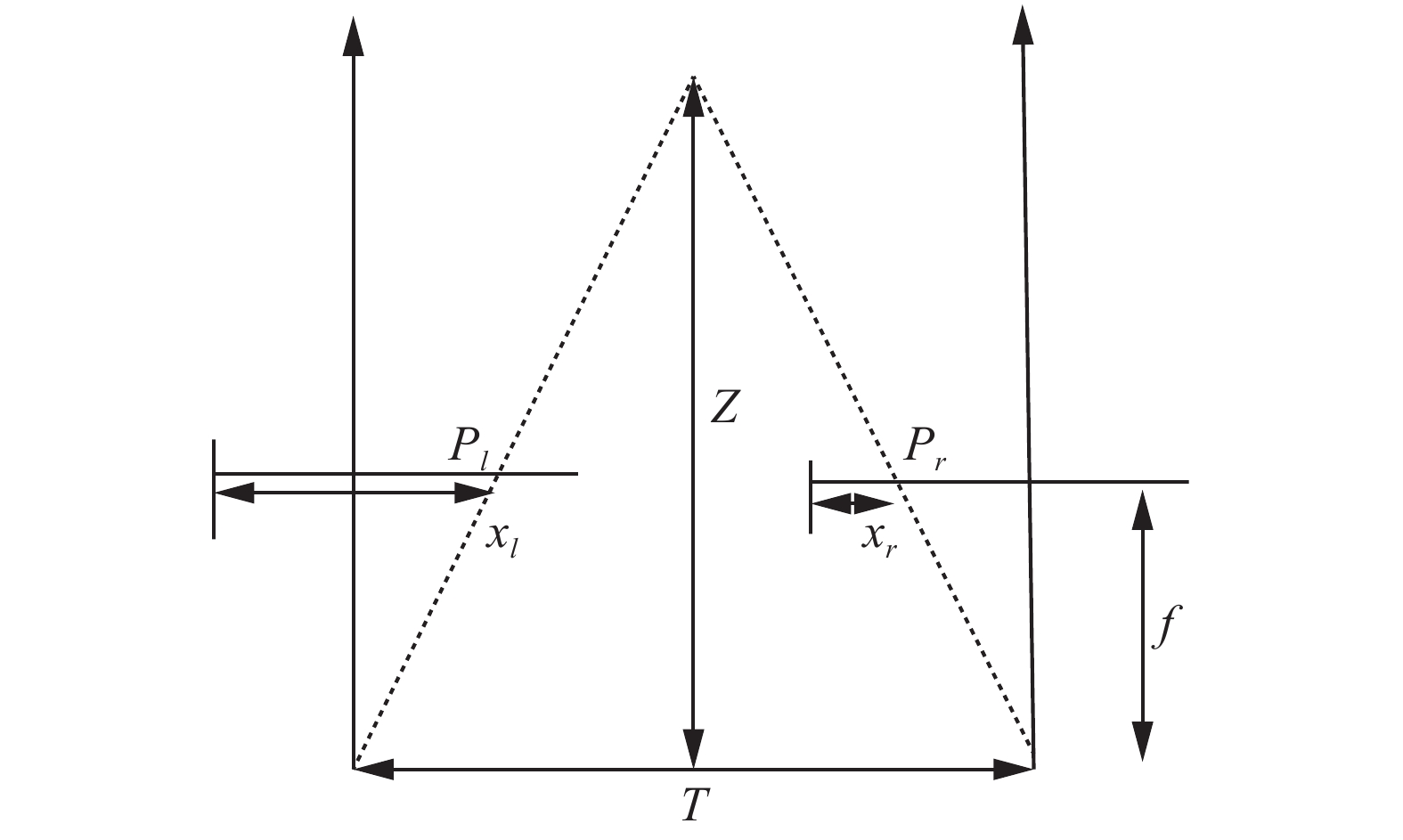Download: 图 1 校正后的立体视觉系统的几何模型 Fig. 1 Rectified stereo vision system

 $\frac{{T - ({x_l} - {x_r})}}{{Z - f}} = \frac{T}{Z}$ (1)

 $Z = \frac{{f \cdot T}}{{{x_l} - {x_r}}} = \frac{{f \cdot T}}{d}$ (2)

2 基于人脸结构的立体匹配算法

2.1 脸部稀疏视差计算

 $D({p_i}) = \sqrt {{{(l_{x_i} - r_{x_i})}^2}} = {\rm{abs}}(l_{x_i} - r_{x_i})$ (3)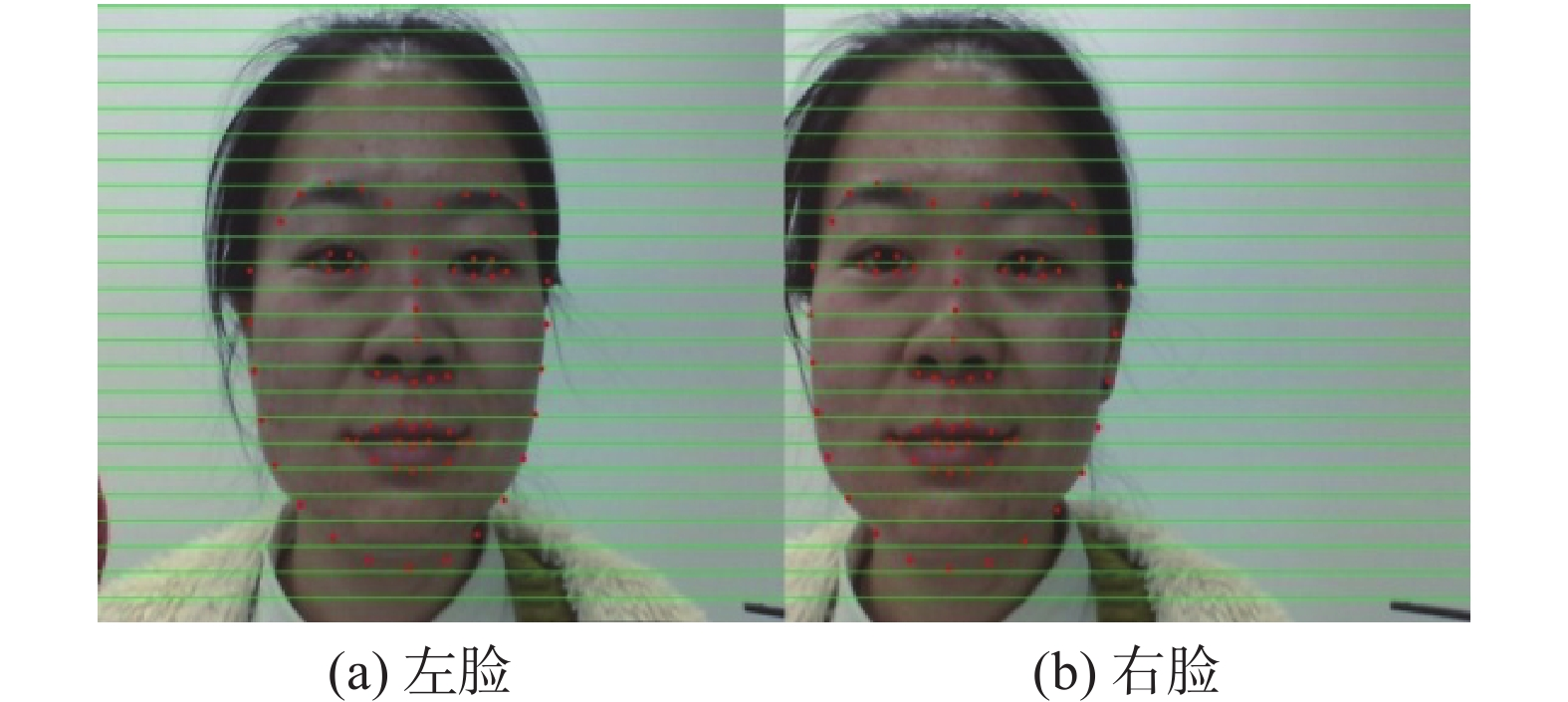Download: 图 2 脸部关键点定位 Fig. 2 Facial landmark
2.2 脸部稠密视差计算

 $p = (1 - u - v) \cdot p_1 + u \cdot p_2 + v \cdot p_3$ (4)

$p$ 点的坐标为 $({p_x},{p_y})$ $p_1$ 点的坐标为 $(p_{1_x},p_{1_y})$ $p_2$ 点的坐标为 $(p_{2_x},p_{2_y})$ $p_3$ 点的坐标为 $(p_{3_x},p_{3_y})$ ，它们满足式(5)的关系，此时就能解出uv参数。

 $\begin{array}{c}{p_x} = (1 - u - v) \cdot p_{1_x} + u \cdot p_{2_x} + v \cdot p_{3_x}\\{p_y} = (1 - u - v) \cdot p_{1_y} + u \cdot p_{2_y} + v \cdot p_{3_x}\end{array}$ (5)

 $D(p) = (1 - u - v) \cdot D(p_1) + u \cdot D(p_2) + v \cdot D(p_3)$ (6)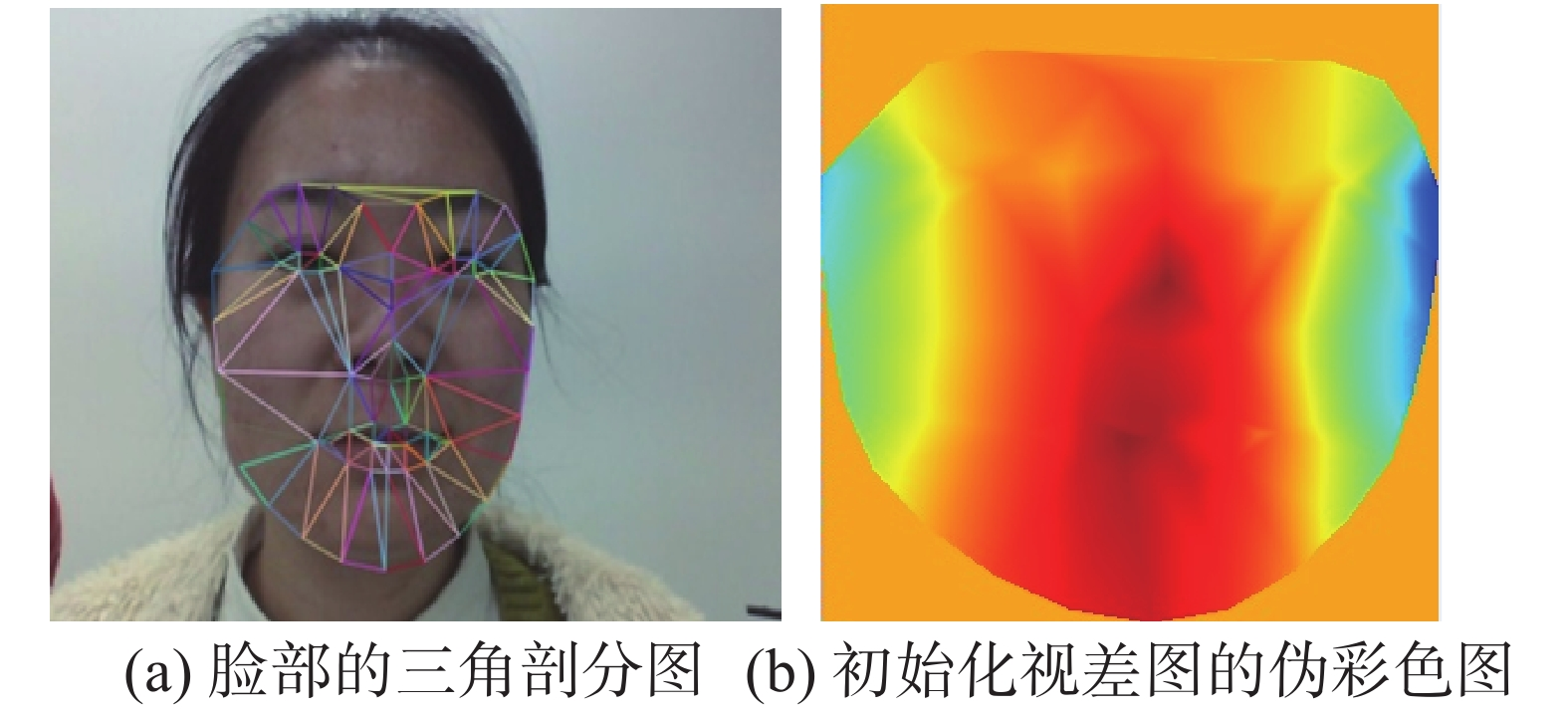Download: 图 3 脸部的三角剖分及视差图的伪彩色图集合 Fig. 3 Face triangulation and Pseudo color image of disparity maps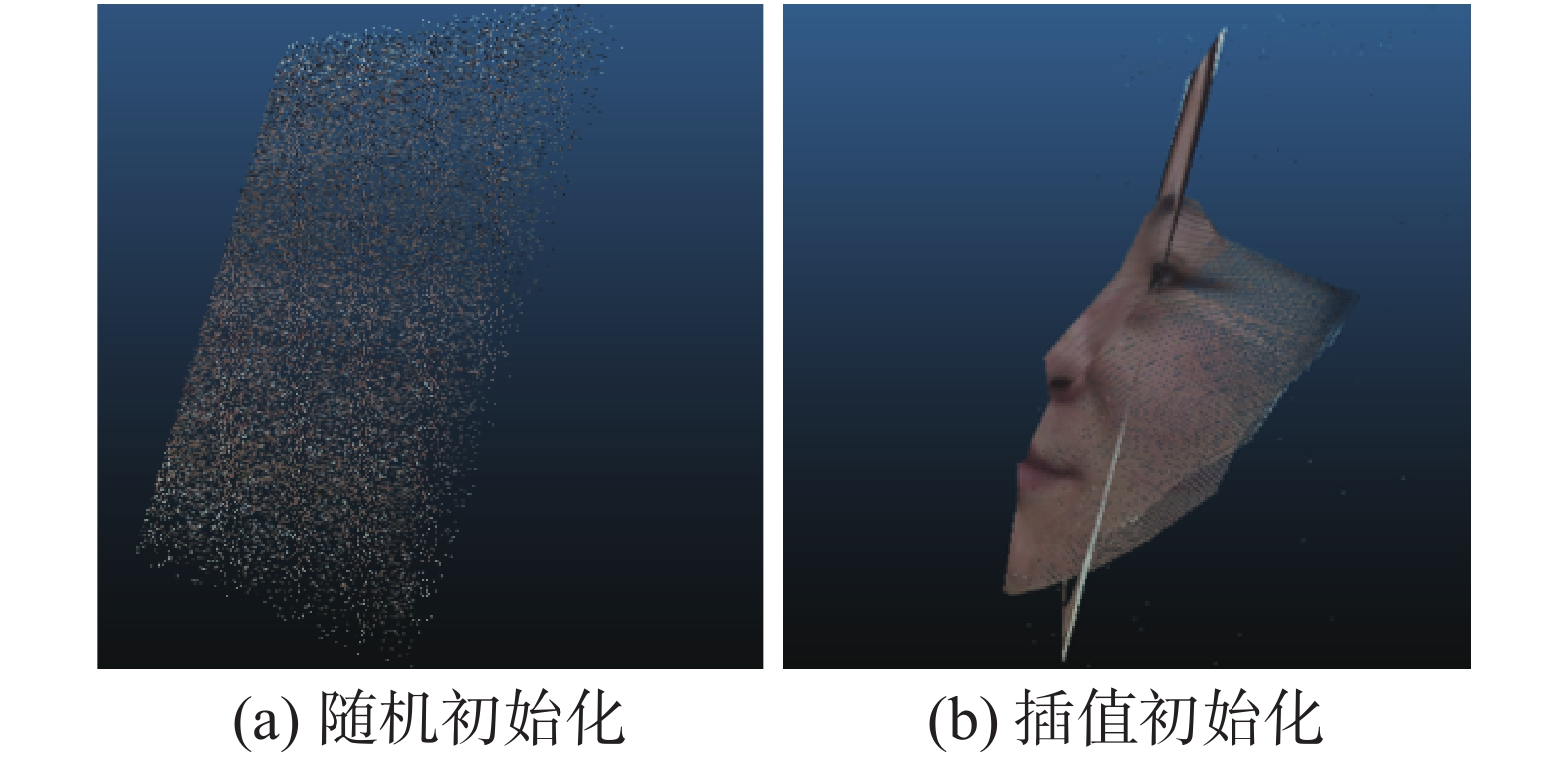Download: 图 4 脸部三维效果图 Fig. 4 Face 3-D renderings
2.3 基于PatchMatch算法的人脸三维结构优化

 $m(p,f) = \sum\limits_{q \in {W_p}}^{} {w(p,q) \cdot \rho (q,q - {d_q})}$ (7)

 $\begin{array}{c}{a_f} = - \displaystyle\frac{{{n_x}}}{{{n_z}}},{b_f} = - \displaystyle\frac{{{n_y}}}{{{n_z}}},{c_f} = \displaystyle\frac{{{n_x}{x_0} + {n_y}{y_0} + {n_z}{z_0}}}{{{n_z}}}\end{array}$ (8)

$\rho (p,q)$ 代表像素pq之间的匹配代价。定义为: $\rho (p,q) = H({\rm{census}}(p) - {\rm{census}}(q))$ 。其中， ${\rm{census}}(p)$ ${\rm{census}}(q)$ 分别代表对像素 $p$ $q$ 进行census编码，然后计算编码后的哈明距离，以此值作为最终的匹配代价。

 $w(p,q) = {{\rm{e}}^{\frac{{ - \left\| {{{{I}}_p} - {{{I}}_q}} \right\|}}{\gamma }}}$ (9)

 ${f_p} = \mathop {\arg \min m(p,f)}\limits_{f \in F}$ (10)

f代表所有的视差平面，所对应的解是无穷多的。通过下面的方式找到较优的平面参数。

3 实验结果及分析

3.1 数据库1评估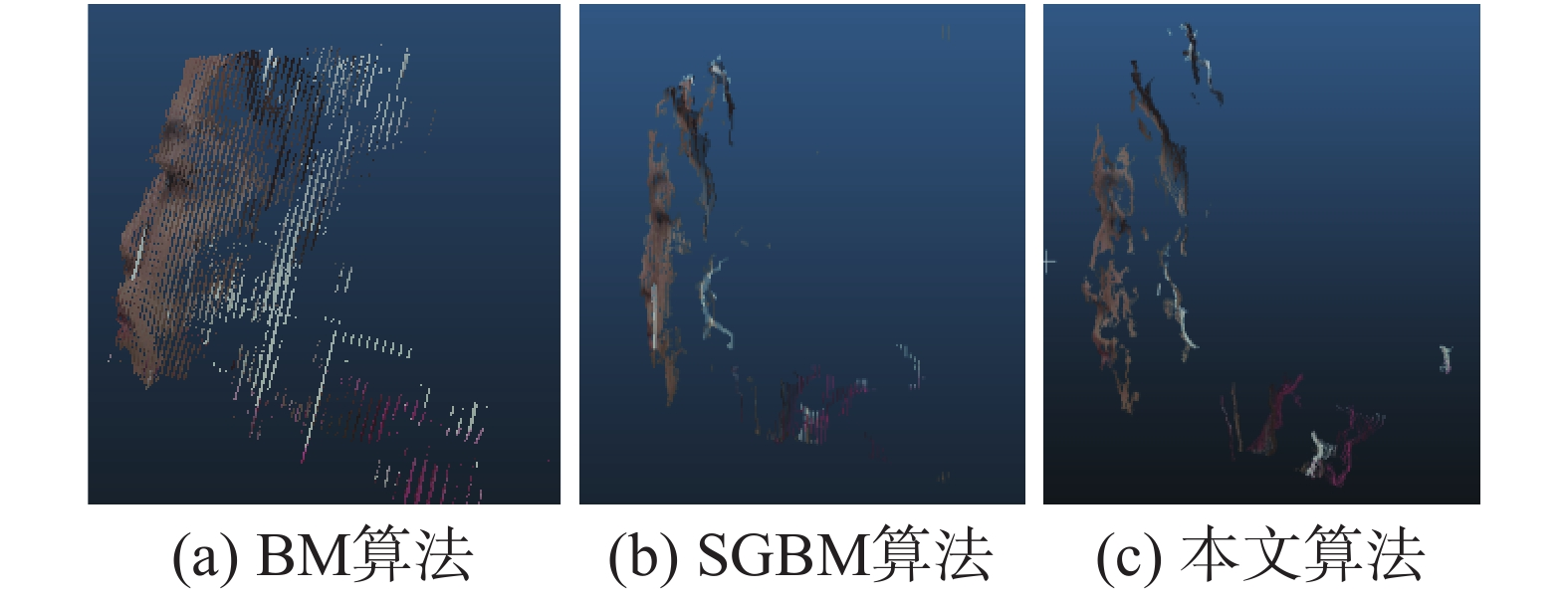Download: 图 5 不同算法对人脸的三维重建效果 Fig. 5 Face 3-D renderings on different algorithms

3.2 Bosphorus数据库评估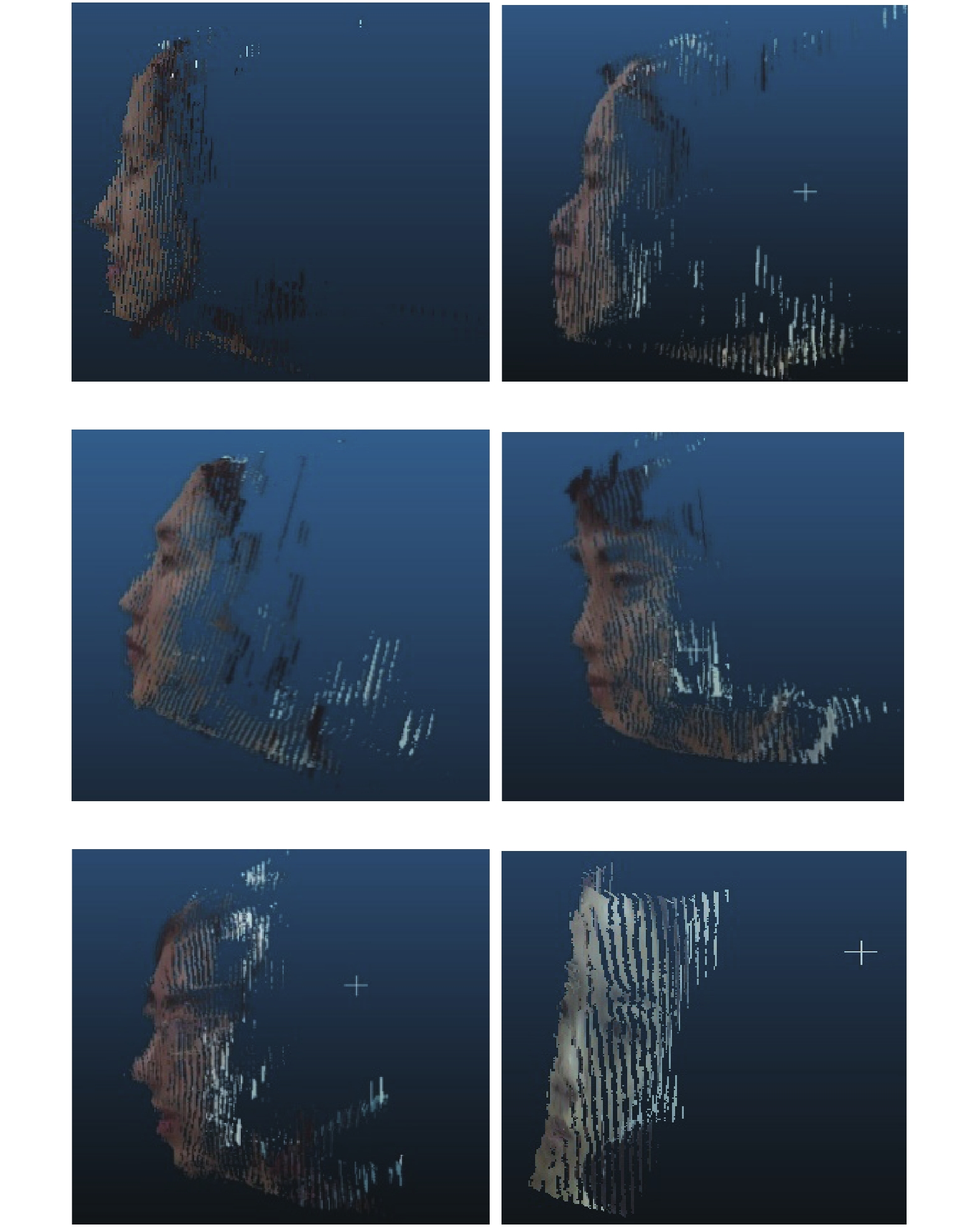Download: 图 6 不同人物的人脸深度图 Fig. 6 Face 3-D renderings on different person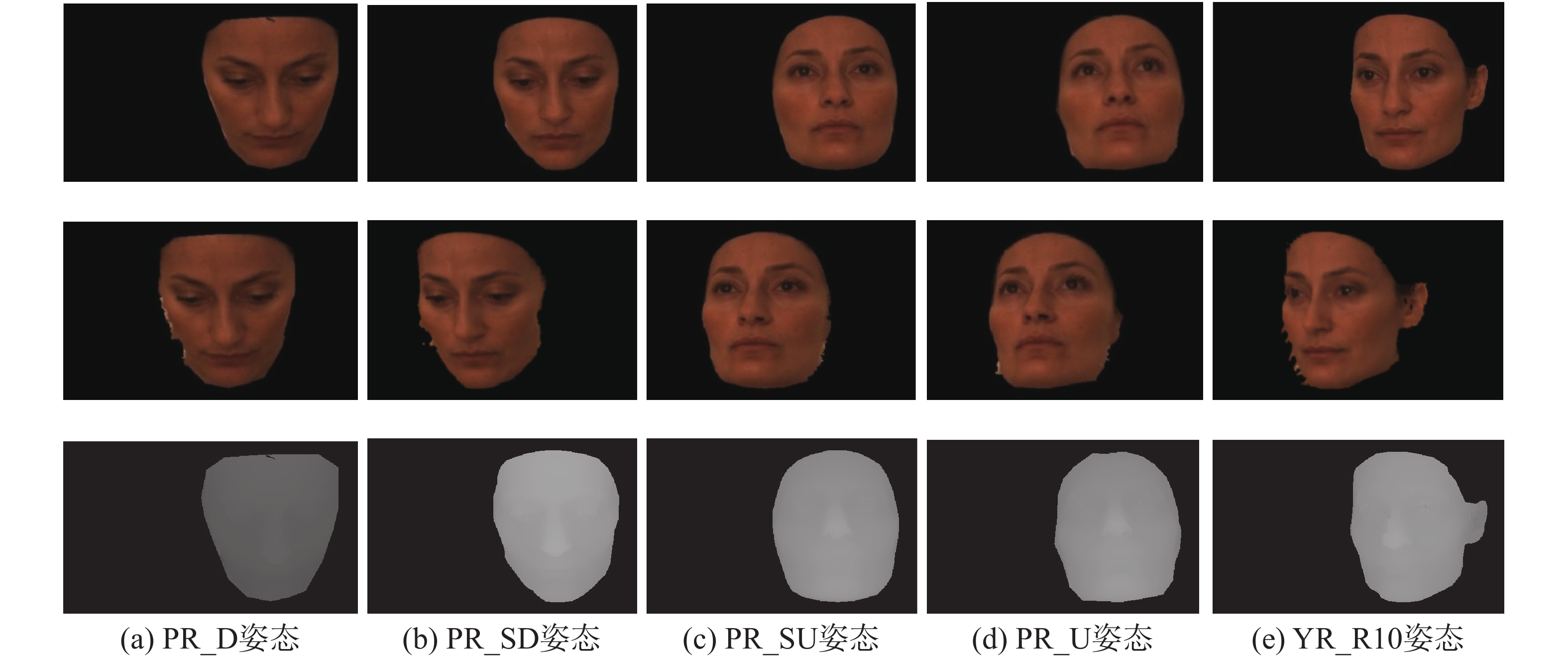Download: 图 7 bs003人物不同姿态 Fig. 7 Different poses of bs003
3.2.1 定性分析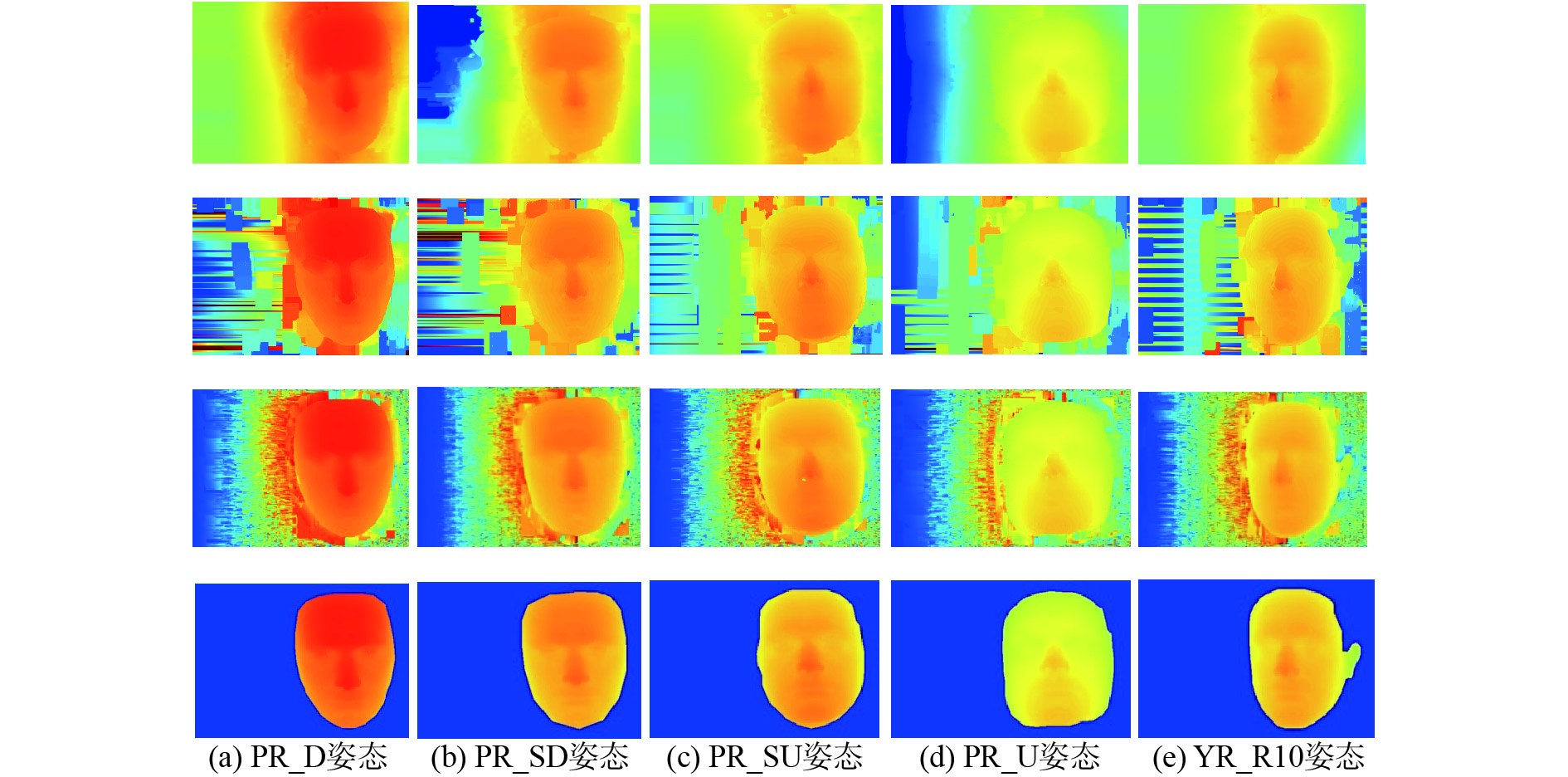Download: 图 8 不同方法在不同姿态下的视差图效果 Fig. 8 Disparity renderings of different face pose on different methods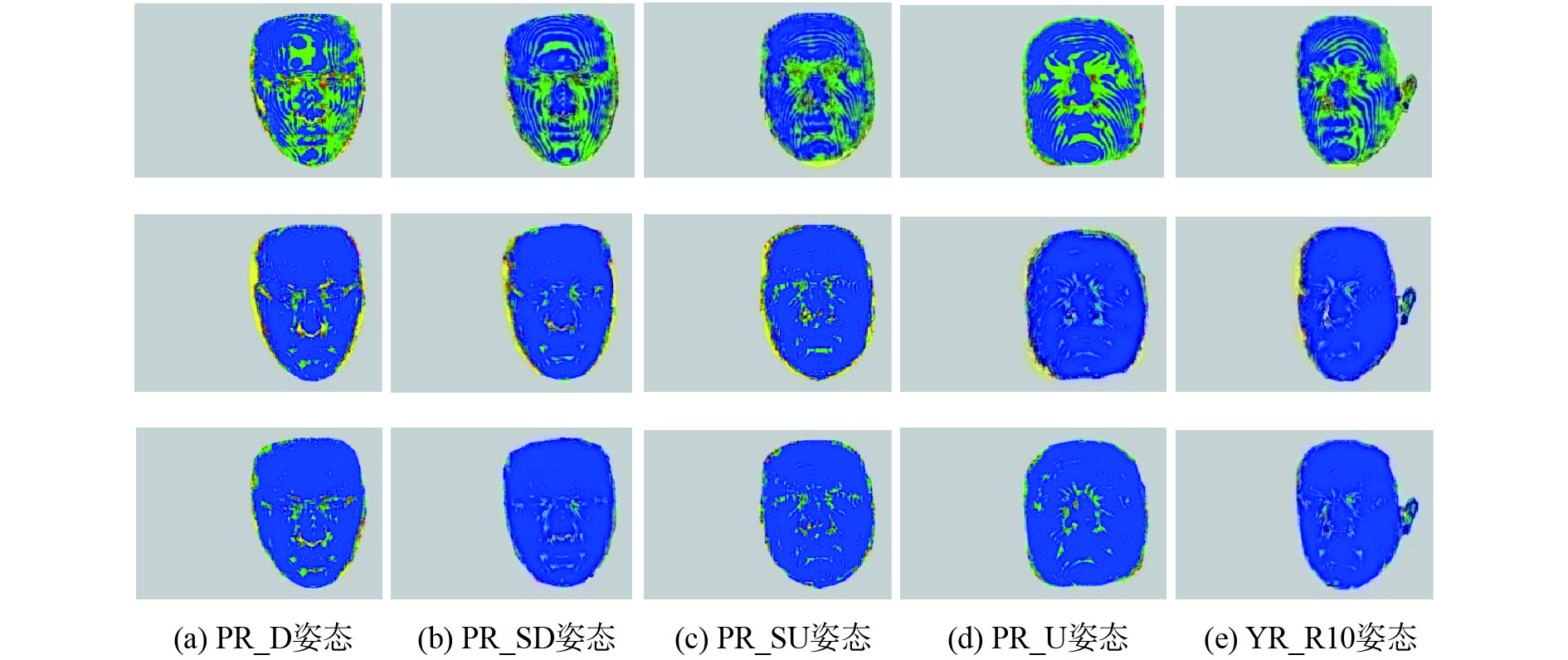Download: 图 9 不同方法在不同姿态下的脸部误差分布图 Fig. 9 Error map of different face pose on different methods
3.2.2 定量分析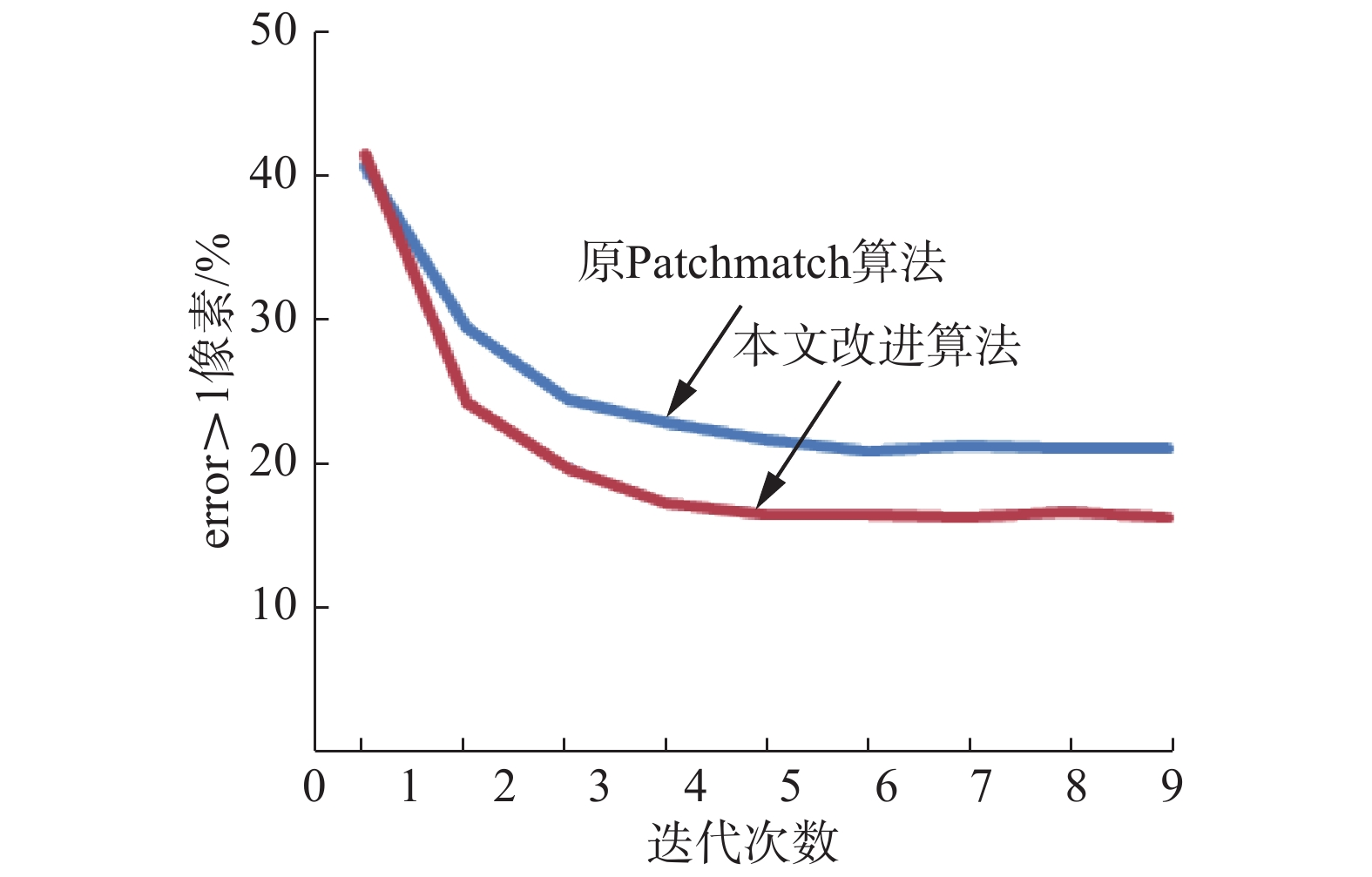Download: 图 10 PatchMatch与本文方法的错误率比较 Fig. 10 Error rate of PatchMatch and proposed method

 ${\rm{PBM}} = \frac{1}{{np}} \times \sum\nolimits_{(x,y)} {D(x,y)}$ (11)

 $D(x,y) = \left\{ \begin{array}{l}\!\!\! 0, \quad \left| {{\rm{d}}E(x,y) - {\rm{d}}T(x,y) \leqslant \delta_d} \right| \\\!\!\! 1, \quad \text{其他}\end{array} \right.$ (12)表 1 不同方法在人脸不同姿态下的PBM值 Tab.1 PBM of different face pose on different methods %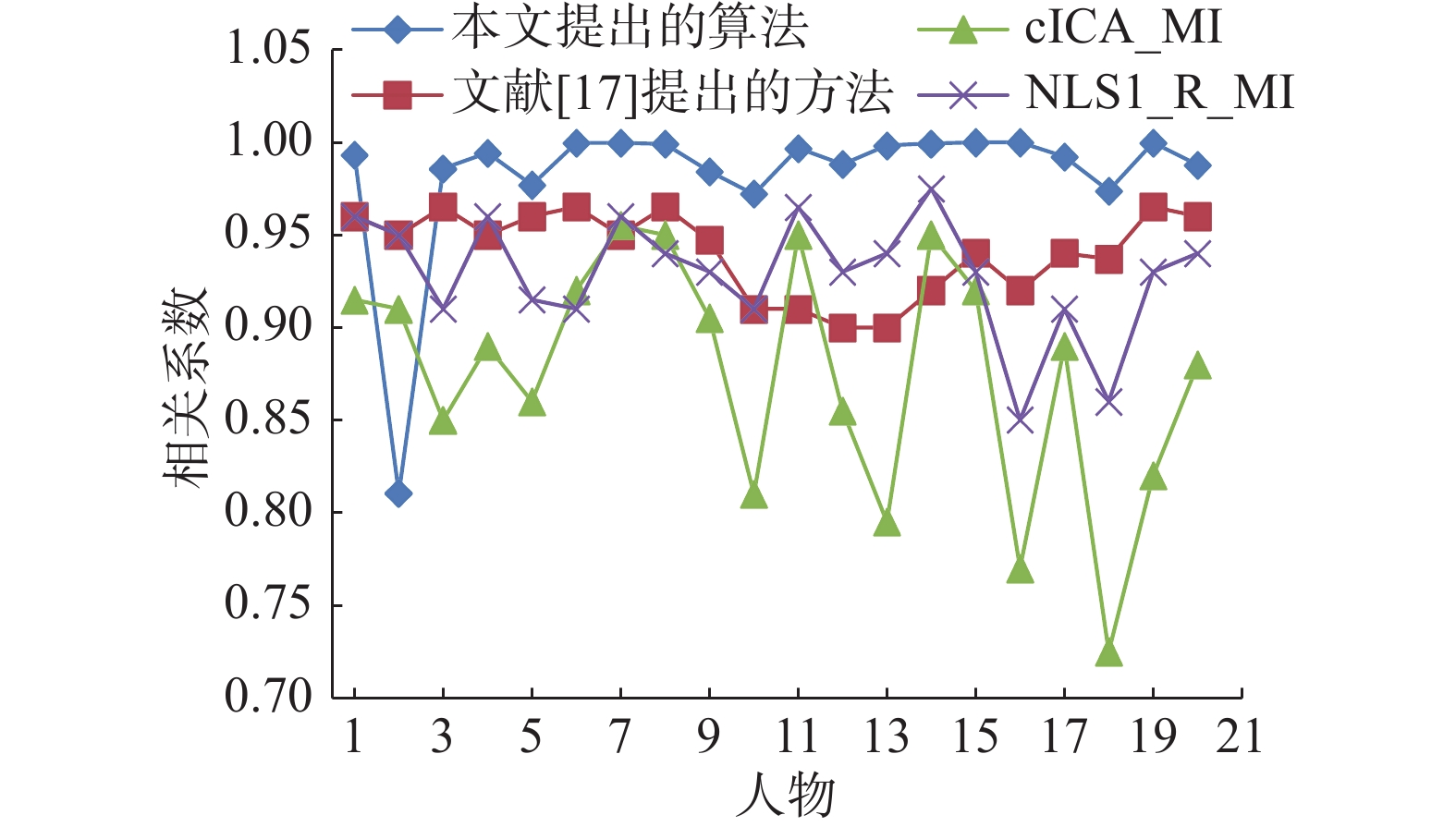Download: 图 11 不同方法对应的前20个人物的相关系数 Fig. 11 The correlation coefficient of the top 20 individuals corresponding to different methods

 $M_z' = \frac{{{M_z} - M_z^{\min }}}{{M_z^{\max } - M_z^{\min }}}$ (13)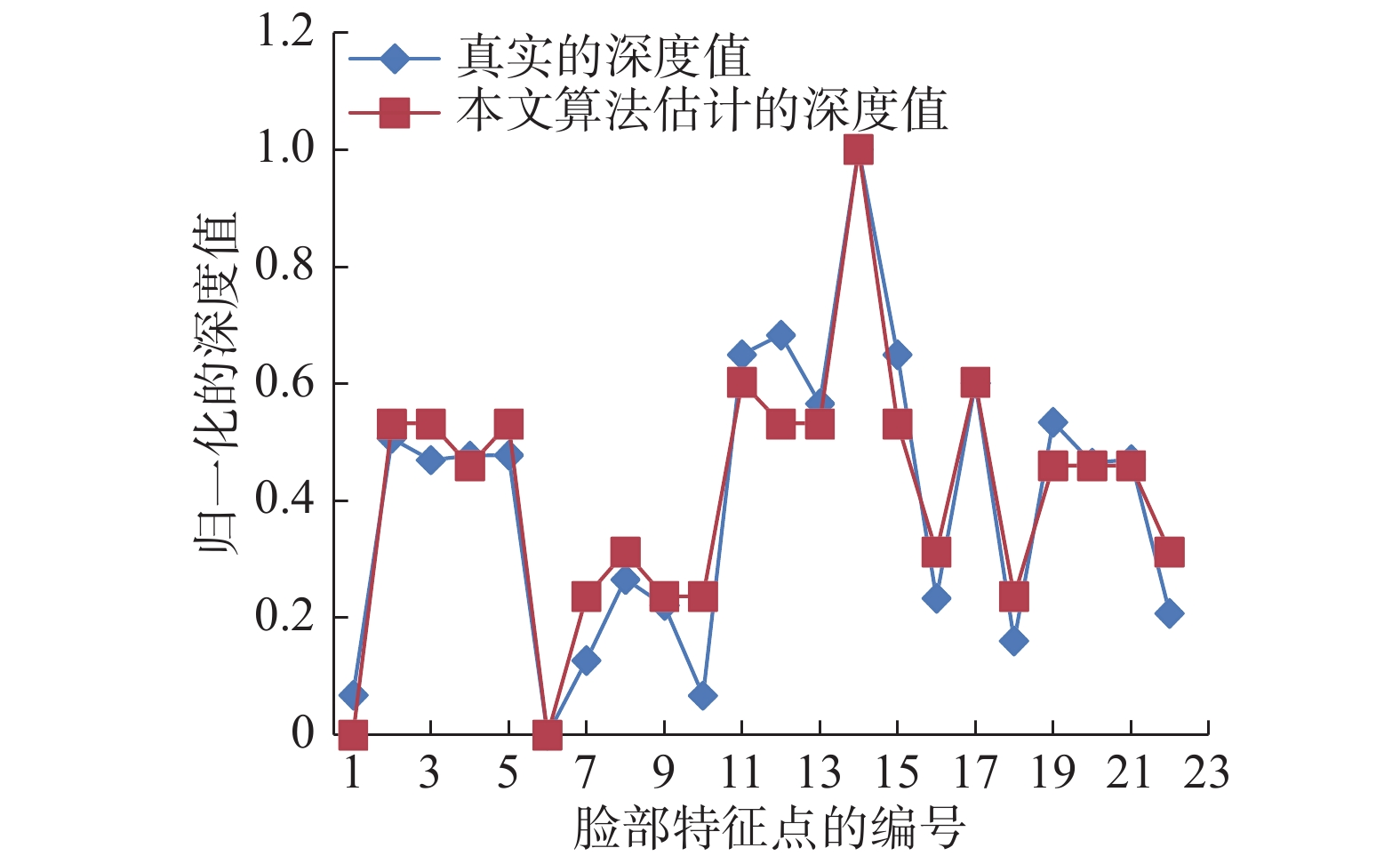Download: 图 12 真实人脸特征点的深度值与本文方法估计的人脸特征点深度值的比较 Fig. 12 Comparison of depth of face key points between ground truth and estimated value by proposed method

4 结束语

  易唐唐, 董朝贤. 基于面部表情GEM和稀疏立方矩阵的三维人脸识别方法[J]. 重庆邮电大学学报: 自然科学版, 2017, 29(2): 257-264. YI Tangtang, DONG Chaoxian. 3D face recognition method based on facial expression generic elastic model and sparse cubic matrix[J]. Journal of Chongqing university of posts and telecommunications: natural science edition, 2017, 29(2): 257-264. (0)  赵军, 赵艳, 杨勇, 等. 基于降维的堆积降噪自动编码机的表情识别方法[J]. 重庆邮电大学学报: 自然科学版, 2016, 28(6): 844-848. ZHAO Jun, ZHAO Yan, YANG Yong, et al. Facial expression recognition method based on stacked denoising auto-encoders and feature reduction[J]. Journal of Chongqing university of posts and telecommunications: natural science edition, 2016, 28(6): 844-848. (0)  ROY-CHOWDHURY A K, CHELLAPPA R. Statistical bias in 3-D reconstruction from a monocular video[J]. IEEE transactions on image processing, 2005, 14(8): 1057-1062. DOI:10.1109/TIP.2005.849775 (0)  KOO H S, LAM K M. Recovering the 3D shape and poses of face images based on the similarity transform[J]. Pattern recognition letters, 2008, 29(6): 712-723. DOI:10.1016/j.patrec.2007.11.018 (0)  AISSAOUI A, AUGUSTE R, YAHIAOUI T, et al. Fast stereo matching method based on optimized correlation algorithm for face depth estimation[C]//Proceedings of International Conference on Image Processing. Orlando, Florida: IEEE Signal Processing Society, 2012: 377–380. (0)  DA F, SUI Y. 3D reconstruction of human face based on an improved seeds-growing algorithm[J]. Machine vision and applications, 2011, 22(5): 879-887. DOI:10.1007/s00138-010-0278-8 (0)  ZHANG R, TSAI P S, CRYER J E, et al. Shape-from-shading: a survey[J]. IEEE transactions on pattern analysis and machine intelligence, 1999, 21(8): 690-706. DOI:10.1109/34.784284 (0)  HORN B K P. Obtaining shape from shading information[C]//Shape from shading. New York: MIT press, 1989: 123–171. (0)  王琨, 郑南宁. 基于SFM算法的三维人脸模型重建[J]. 计算机学报, 2005, 28(6): 1048-1053. WANG Kun, ZHANG Nanning. 3D Face modeling based on SFM algorithm[J]. Chinese journal of computer, 2005, 28(6): 1048-1053. (0)  SUN Z L, LAM K M, GAO Q W. Depth estimation of face images using the nonlinear least-squares model[J]. IEEE transactions on image processing, 2013, 22(1): 17-30. DOI:10.1109/TIP.2012.2204269 (0)  SUN Z L, LAM K M. Depth estimation of face images based on the constrained ICA model[J]. IEEE Transactions on information forensics and security, 2011, 6(2): 360-370. DOI:10.1109/TIFS.2011.2118207 (0)  CHOI J, MEDIONI G, LIN Y, et al. 3D face reconstruction using a single or multiple views[C]//Proceedings of International Conference on Pattern Recognition 2010. Istanbul : IEEE Conference Publications, 2010: 3959–3962. (0)  LIU F, ZENG D, ZHAO Q, et al. Joint face alignment and 3D face reconstruction[C]// Proceedings of European Conference on Computer Vision. Springer International Publishing, 2016: 545–560. (0)  HUBER P, HU G, TENA R, et al. A multiresolution 3D Morphable Face Model and fitting framework[C]//Proceedings of the 11th International Joint Conference on Computer Vision, Imaging and Computer Graphics Theory and Applications. [S.l.], 2016: 151–155. (0)  HIRSCHMULLER H. Improvements in real-time correlation-based stereo vision[C]//Proceedings of Stereo and Multi-Baseline Vision. Kauai, Hawaii: IEEE Computer Society, 2001: 0141. (0)  HIRSCHMULLER H. Stereo processing by semiglobal matching and mutual information[J]. IEEE transactions on pattern analysis and machine intelligence, 2008, 30(2): 328-341. DOI:10.1109/TPAMI.2007.1166 (0)  ZHANG C, LI Z, CHENG Y, et al. Meshstereo: lobal stereo model with mesh alignment regularization for view interpolation[C]//Proceedings of International Conference on Computer Vision. Santiago, Chile: the IEEE, 2015: 2057–2065. (0)  AISSAOUI A, MARTINET J, DJERABA C. Rapid and accurate face depth estimation in passive stereo systems[J]. Multimedia tools and applications, 2014, 72(3): 2413-2438. DOI:10.1007/s11042-013-1556-z (0)  BLEYER M, RHEMANN C, ROTHER C. PatchMatch stereo-stereo matching with slanted support windows[C]//Proceedings of British Machine Vision Conference. Dundee: Warwick Print, 2011, 11: 1–11. (0)  KAZEMI V, SULLIVAN J. One millisecond face alignment with an ensemble of regression trees[C]//Proceedings of Computer Vision and Pattern Recognition. Boston, Massachusetts: IEEE, 2014: 1867–1874. (0)  ZABIH R, WOODFILL J. Non-parametric local transforms for computing visual correspondence[M]//Computer Vision—ECCV'94. Springer Berlin Heidelberg, 1994: 151–158. (0)  SAVRAN A, ALYÜZ N, DIBEKLIOĞLU H, et al. Bosphorus database for 3D faceanalysis[C] //Proceedings of European Workshop on Biometrics and Identity Management. Springer Berlin Heidelberg, 2008: 47–56. (0)